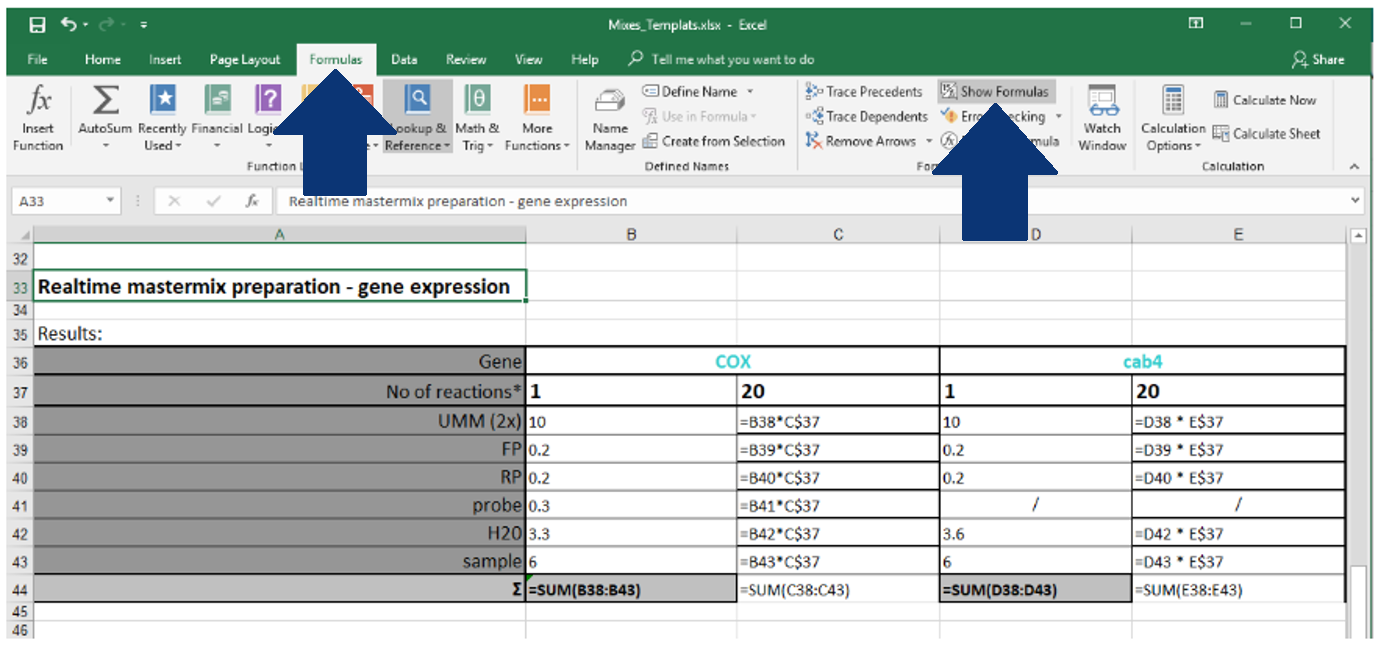1. Customer Support
2. Getting Started
3. How to get most out of SciNote

# Using math operations and formulas in SciNote tables

Tables that are created under the section Table in a protocol step or under Results in SciNote support basic math operations just as Excel spreadsheets do, but with some exceptions. The functions that can be used in SciNote tables are the following:

 Function name Type and description DATE function Date and time:    Returns the serial number of a particular date DATEVALUE function Date and time:    Converts a date in the form of text to a serial number DAY function Date and time:    Converts a serial number to a day of the month DAYS function Date and time:    Returns the number of days between two dates EDATE function Date and time:    Returns the serial number of the date that is the indicated number of months before or after the start date AND function Logical:    Returns TRUE if all of its arguments are TRUE FALSE function Logical:    Returns the logical value FALSE IF function Logical:    Specifies a logical test to perform NOT function Logical:    Reverses the logic of its argument OR function Logical:    Returns TRUE if any argument is TRUE TRUE function Logical:    Returns the logical value TRUE ABS function Math and trigonometry:    Returns the absolute value of a number INT function Math and trigonometry:    Rounds a number down to the nearest integer LN function Math and trigonometry:    Returns the natural logarithm of a number LOG function Math and trigonometry:    Returns the logarithm of a number to a specified base LOG10 function Math and trigonometry:    Returns the base-10 logarithm of a number PI function Math and trigonometry:    Returns the value of pi POWER function Math and trigonometry:    Returns the result of a number raised to a power ROUND function Math and trigonometry:    Rounds a number to a specified number of digits SQRT function Math and trigonometry:    Returns a positive square root SUM function Math and trigonometry:    Adds its arguments SUMIF function Math and trigonometry:    Adds the cells specified by a given criteria SUMIFS function Math and trigonometry:    Adds the cells in a range that meet multiple criteria TRUNC function Math and trigonometry:    Truncates a number to an integer AVERAGE function Statistical:    Returns the average of its arguments AVERAGEA function Statistical:    Returns the average of its arguments, including numbers, text, and logical values AVERAGEIF function Statistical:    Returns the average (arithmetic mean) of all the cells in a range that meet a given criteria CORREL function Statistical:    Returns the correlation coefficient between two data sets COUNT function Statistical:    Counts how many numbers are in the list of arguments COUNTA function Statistical:    Counts how many values are in the list of arguments COUNTIF function Statistical:    Counts the number of cells within a range that meet the given criteria COUNTIFS function Statistical:    Counts the number of cells within a range that meet multiple criteria INTERCEPT function Statistical:    Returns the intercept of the linear regression line MAX function Statistical:    Returns the maximum value in a list of arguments MAXA function Statistical:    Returns the maximum value in a list of arguments, including numbers, text, and logical values MEDIAN function Statistical:    Returns the median of the given numbers MIN function Statistical:    Returns the minimum value in a list of arguments MINA function Statistical:    Returns the smallest value in a list of arguments, including numbers, text, and logical values SLOPE function Statistical:    Returns the slope of the linear regression line STDEV.P function Statistical:    Calculates standard deviation based on the entire population STDEV.S function Statistical:    Estimates standard deviation based on a sample T.TEST function Statistical:    Returns the probability associated with a Student's t-test CHAR function Text:    Returns the character specified by the code number CODE function Text:    Returns a numeric code for the first character in a text string CONCATENATE function Text:    Joins several text items into one text item EXACT function Text:    Checks to see if two text values are identical

PRODUCT function can't be used in SciNote tables, but you can use * instead.

Furthermore, when copy-pasting the numbers from the Excel file to the SciNote table, you need to open the view Show formulas in an Excel file first (see the image below) and then copy the content and paste it into the SciNote table using CTRL + V keys.Please note that formulas are based on the position of cells, so if you copy-paste Excel table cells from C37:C44 to SciNote table cells A1:A8 the formulas won't function because of different cell positions.

Tables that are created in the rich text fields do not support formulas, they do, however, have formatting options.